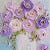# Functions in Java

The assert. assert equals method asserts that two objects are equal. If they are not, an Assertion Error without a message is thrown. If expected and actual are null, they are considered equal. AssertEquals() internally uses the equals() function to validate if two objects are equal. Hence, the below test passes as it validates if the object values are equal.

`package` `ordertests.com;`

`import` `static` `org. junit. Assert. *;`

`import` `org. junit. Test;`

`public` `class` `Assertion2 {`

`public` `void` `test() {`

`String s1 = new` `String("HELLO ......my name is darshana");`

`String s2 = new` `String("HELLO ,my name is dash");`

`assertEquals ("the values are not equal", s1, s2);`

`}`

`}`

--

--

# Exception Handling in Java

what do u mean by exception? so… Exception is an error handling. Generally, these errors are runtime errors. Exception handling is an effective way of handling runtime errors so the flow of the application can be maintained. there are two types of exception handling that are checked and unchecked. another one is an error that can be also considered as an exception Handling. the classes that directly inherit throwable classes excess for runtime so this exception is identified at compile time and we can say vice versa in an unchecked exception handling. also, there are approaches for handling like try and catch, throw and throws and finally.

For Example:
public class check {
public static void main(String[] args);
try{
int x = 10, y = 0, z;
z = x / y;
System.out.println(z);

}
catch(Exception e)

{
System.out.println(e);
}
}

--

--

# Pandas in Python

we can say panda is a library in python which is used to analyze the data. It includes the functions like analyzing, cleaning manipulating, and Exploring data. Pandas are used to analyze big data and make conclusions based on statistical theories. they are capable of cleaning the messy data and making them ready to read and relevant. Relevant data is very important in data science. Data Science is a branch of computer science where we study how to store, use and analyze data for deriving information from it. Pandas will also give information about what is a correlation between two columns? , what is the average, minimum and maximum value?.

For example:

import pandas

my_dataset = {‘cars’: [“BMW”, “Volvo”, “Ford”], ‘passings’: [3, 7, 2]}

my_var= pandas.DataFrame(mydataset)

print(my_var)

--

--

# Python Functions

A function is a block of code which only runs when it is called. You can pass data, known as parameters, into a function. A function can return data as a result. In Python, function can be defined by using def keyword. To call the function, Function name with parenthesis is used. Information can be passed into functions as arguments. Arguments are written after the function name, inside the parentheses. You can add as many arguments as you want, just separate them with a comma. Arguments are often shortened to args in Python.

For example:

def my_function(***child):
print(“His last name is “ + child[“lname”])

my_function(fname = “Darshana”, lname = “Toke”)

we can pass list as argument in python function.

def my_function(food):
for x in food:
print(x)

fruits = [“apple”, “banana”, “cherry”]

my_function(fruits)

--

--